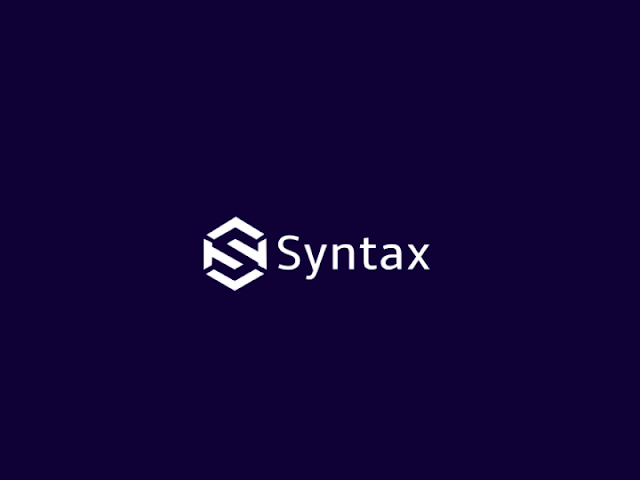# Write a java program with object will do the sum of 1 + 1/4 + 1/7 + 1/9 + ........ + 1/n

import java.util.Scanner;

class Question_18{

public void series(int n){ //5

System.out.print("\n\n Series : ");

System.out.print("1 + ");

for(int i=2;i<=n;i++){

System.out.print("1/"+(i*i)+" + ");

}

}

public static void main(String args[]){

Question_18 a=new Question_18();

Scanner scan=new Scanner(System.in);

System.out.print("Enter N Value for(1+1/4+1/9....1/N) : "); //5

int n=scan.nextInt();

a.series(n);

}

}

Draw a ring in C graphics

Text generation program in C graphics